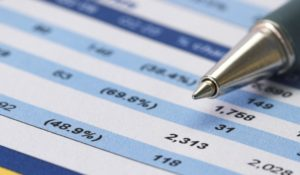# Need for Depreciation and Factors Affecting Amount of Depreciation

The compilation of these Depreciation, Provision and Reserves Notes makes students exam preparation simpler and organised.

## Need for Depreciation and Factors Affecting Amount of Depreciation

When an accountant prepares the accounts of a firm and jots down the amount of depreciation, he brings down the potential gains of the firm. The amount of depreciation is an expense for an entity. Thus, it is imperative to make the correct and accurate calculations. Let us understand what is depreciation and why we need to provide for it.

### Need to Provide Depreciation

The depreciation needs to be provided because an asset is bound to undergo wear and tear over a period of time. This reduces the working capacity and effectiveness of the asset. Hence, this should reflect the value of the asset, at which it is carried in the books of accounts.

Also, every asset becomes obsolete over a period of time, as new technology and innovation take over. The value of the asset will hence decrease over time and this must be accounted for.

Moreover, in order to comply with the matching principle of accounts, it is ideal to provide depreciation. The Matching principle says that the expense of a period must be recognized in the same period in which we recognize its revenue. So an asset which generates income must be depreciated as per the given provisions.

### Factors Affecting Amount of DepreciationThe amount of depreciation is impacted by a number of factors. Let us take a look at some of them. There are four main factors to consider when calculating the depreciation expense are as follows:

• The cost of the asset.
• The estimated salvage value of the asset. Salvage value (also called residual value) is the amount of money that the company expects to recover, less the disposal costs, on the date the asset is scrapped, sold, or traded in.
• The estimated useful life of the asset. Useful life refers to the window of time that a company plans to use an asset. Useful life can be expressed in years, months, working hours, or units produced.
• Obsolescence should be considered when determining an asset’s useful life and will affect the calculation of depreciation. For example, a machine capable of producing units for 20 years may be obsolete in six years; therefore, the asset’s useful life is six years in this case.

A company is free to make use of the most appropriate depreciation method for its business operations. Accounting theory suggests that companies should make use of a depreciation method that closely reflects the company’s’ economic circumstances. Thus, companies can choose a method that allocates the asset cost to accounting periods according to benefits received from the use of the asset.

Most companies use the straight-line method for financial reporting purposes, but they may also use different methods for different assets. The most important criteria to follow is to Use a depreciation method that allocates asset cost to accounting periods in a systematic and rational manner.

Example:

Question:
How do factors affecting depreciation impact the amount of depreciation?